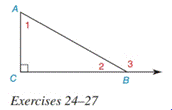Chapter 2.4, Problem 25E### Elementary Geometry for College St...

6th Edition
Daniel C. Alexander + 1 other
ISBN: 9781285195698

#### Solutions

Chapter
Section### Elementary Geometry for College St...

6th Edition
Daniel C. Alexander + 1 other
ISBN: 9781285195698
Textbook Problem
1 views

#In Exercises 25 to 27 , see the figure for exercise 24 . Given: m ∠ 1 = x , m ∠ 2 = y , m ∠ 3 = 3 x Prove: x and y

To determine

To find:

The value of x and y.

Explanation

Given:

m1=x,

m2=y

And,

m3=3x

Figure (1)

Concept:

(1) Sum of acute angles in a right triangle is equal to 90°.

(2) Sum of linear pair of angles is equal to 180°.

Calculation:

Equate the sum of acute angles in a right triangle to 90°.

m1+m2=90°(1)

Substitute x for m1, and y for m2 in equation (1).

x+y=90°

Equate the sum of linear pair of angles to 180°

### Still sussing out bartleby?

Check out a sample textbook solution.

See a sample solution

#### The Solution to Your Study Problems

Bartleby provides explanations to thousands of textbook problems written by our experts, many with advanced degrees!

Get Started